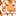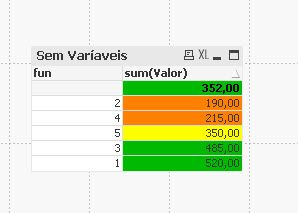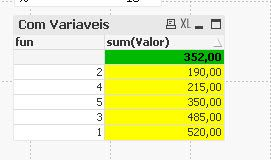# QlikView App Dev

Discussion Board for collaboration related to QlikView App Development.

Announcements
Support Cases coming to Qlik Community Oct. 4! Start chats, open cases, explore resources: READ DETAILS
cancel
Showing results for
Did you mean:Specialist

## I'm not able to use If with colors in variables.

I need to place the colors according to the color scheme. But with variables.

=if(Column(1) <= 300,RGB(255,128,0),
if(Column(1) >= 301 and Column(1) <= 352,RGB(255,255,0),
if(Column(1) >= 353,RGB(0,185,0),RGB(255,255,255))))

No Variables:  Works in Color 😀vMedia: num(sum(Valor)/Count(DISTINCT fun),' #.##0,00')

vMedia+10: num((sum(Valor) / Count(DISTINCT fun) * vValor / 100) + (sum(Valor) / Count(DISTINCT fun)),' #.##0,00')

vMedia-10:num((-sum(Valor) / Count(DISTINCT fun)) * vValor / 100) - num(-(sum(Valor) / Count(DISTINCT fun)),'#.##0,00')

Set Analysis:

=if(Column(1) <= \$(vMedia-10),RGB(255,128,0),
if(Column(1) >= \$(vMedia) and Column(1) <= \$(vMedia+10),RGB(255,255,0),
if(Column(1) >= \$(vMedia+10),RGB(0,185,0),RGB(255,255,255))))

With Variables😂Neves
1 Solution

Accepted SolutionsMVP

@Ribeiro  see the attached.  You may need to change the conditions based on your variable values because what you have entered manually is different than what comes from variable.

2 RepliesMVP

@Ribeiro  see the attached.  You may need to change the conditions based on your variable values because what you have entered manually is different than what comes from variable.Specialist
Author

😀😁Thank you very much.

NevesCommunity Browser